# Introduction

This vignette reproduces results and figures in

Kopp-Schneider, A., Wiesenfarth, M., Witt, R., Edelmann, D., Witt, D. and Abel, U. (2018). Monitoring futility and efficacy in phase II trials with Bayesian posterior distributions - a calibration approach. Biometrical Journal, to appear.

Note that most figures are also shown in the interactive shiny app using BDP2workflow()

# Parameters

First we set the parameters as in the paper. Notation is identical as in the paper: p0, p1, pF, pE, cF, cE as in the paper, shape1F, shape2F are the parameters for the prior for futility, and shape1E, shape2E are the parameters for the prior for efficacy.

library(BDP2)
p0=0.12
p1=0.3
pF=0.3
pE=0.12
shape1F=0.3
shape2F=0.7
shape1E=0.12
shape2E=0.88
cF=0.01
cE=0.9

# Figure 1

Figure 1: Posterior distribution of response probability used for futility stop decision: the trial will be stopped for futility if the coloured area is $$< c_F$$ (left panel). Posterior distribution of response probability used for efficacy calling: the trial will be called efficaceous if the coloured area is $$\geq c_E$$ (right panel). The Figure also shows the choice of $$p_F=p_1$$ and of $$p_E=p_0$$ as discussed in the actual design of the trial.

##plot PF
plot(function(x) x,0,0.8,add=F,type="n",col="black",xlab="",ylab="",xaxt="n",yaxt="n",ylim=c(0,4),
cex.axis=1.5,cex.lab=1.5,xaxs='i',yaxs='i')
plot(function(x) dbeta(x,shape1F+2,shape2F+8),add=T,col="black",lwd=2,lty=1)
xy <- seq(0.3,1,length=1000)
fxy <- dbeta(xy,shape1F+2,shape2F+8)
xyx <- c(1,0.3,xy)
yyx <- c(0.0001,0.0001,fxy)
polygon(xyx,yyx,col='red')
lines(c(0.3,0.3),c(0,dbeta(0.3,shape1F+2,shape2F+8)),lwd=3)
axis(side=1,at=c(0.12,0.3),label=c(expression(p[E]),expression(p[F])),cex.axis=1.5)
axis(side=1,at=c(0.12,0.3),label=c(expression(paste("=",p)),expression(paste("=",p))),cex.axis=1.5,line=1.5,lty=0)
text(0.65,0.7,labels=expression(paste("P(p > ",p[F],"| Data)")),cex=1.5)
lines(c(0.4,0.5),c(0.5,0.7),lwd=3)

##plot PE
plot(function(x) x,0,0.8,add=F,type="n",col="black",xlab="",ylab="",xaxt="n",yaxt="n",ylim=c(0,4),
cex.axis=1.5,cex.lab=1.5,xaxs='i',yaxs='i')
plot(function(x) dbeta(x,shape1E+2,shape2E+8),add=T,col="black",lwd=2,lty=1)
xy <- seq(0.12,1,length=1000)
fxy <- dbeta(xy,shape1E+2,shape2E+8)
xyx <- c(1,0.12,xy)
yyx <- c(0.0001,0.0001,fxy)
polygon(xyx,yyx,col='green')
lines(c(0.12,0.12),c(0,dbeta(0.12,shape1E+2,shape2E+8)),lwd=3)
axis(side=1,at=c(0.12,0.3),label=c(expression(p[E]),expression(p[F])),cex.axis=1.5)
axis(side=1,at=c(0.12,0.3),label=c(expression(paste("=",p)),expression(paste("=",p))),cex.axis=1.5,line=1.5,lty=0)
text(0.65,0.7,labels=expression(paste("P(p > ",p[E],"| Data)")),cex=1.5)
lines(c(0.4,0.5),c(0.5,0.7),lwd=3)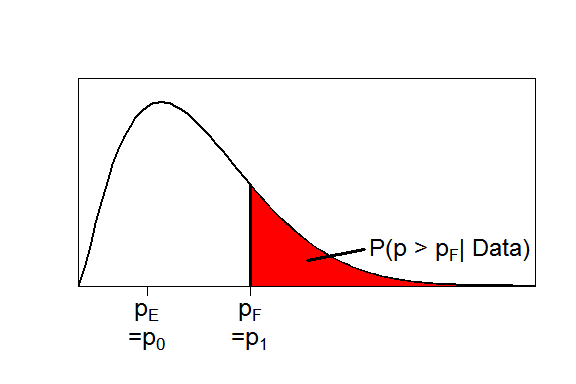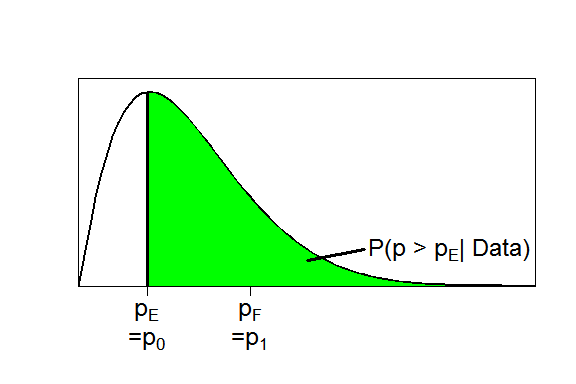# Figure 2

Upper tail function for $$n=20$$, for a design with $$p_F=0.3$$, $$c_F = 0.01$$ and prior distribution Be($$p_F,1-p_F$$) for futility (in red), and $$p_E=0.12$$, $$c_E = 0.9$$ and prior distribution Be($$p_E,1-p_E$$) for efficacy (in green). From the graphs, $$b_F(20)=1$$ and $$b_E(20)=5$$.

n=20

plot(function(x) pbeta(pF,shape1=shape1F+x,shape2=shape2F+n-x,lower.tail = F)-cF,
0,n,ylim=c(-1,1),lwd=3,
xlab="x",ylab=expression(paste("P(p>",p[R],"|x,n = 20) - c")),col="red")

plot(function(x) pbeta(pE,shape1=shape1E+x,shape2=shape2E+n-x,lower.tail = F)-cE,
0,n,ylim=c(-1,1),lwd=3,col="green",add=TRUE)
abline(h=0)
legend("bottomright",legend=c(expression(paste("Futility decision: c=",c[F],", ",p[R],"=",p[F])),
expression(paste("Efficacy decision: c=",c[E],", ",p[R],"=",p[E]))),
lty=1,col = c("red", "green"),cex=1)
abline(v=c(0:20),lty=3,col="grey")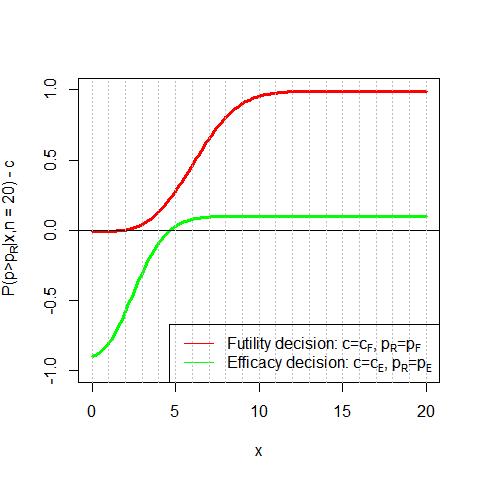# Figure 3

Binomial density with zero responders,$$(1-p)^n$$, for varying $$n$$, evaluated for $$p=p_0=0.12$$ and for $$p=p_1=0.3$$ (left panel). Posterior probabilities $$P(p \geq p_F | 0,n)$$ and $$P(p \geq p_F | 1,n)$$ for varying $$n$$ for $$p_F=0.3$$ with prior distribution Beta($$p_F,1-p_F$$) (right panel).

nmin=4
nmax=15

plotBDP2(x="n",y="Prob0Successes",n=c(nmin,nmax),p0=p0,p1=p1)

plotBDP2(x="n",y="PostProb0or1Successes",n=c(nmin,nmax),pF=pF,shape1F=shape1F,shape2F=shape2F)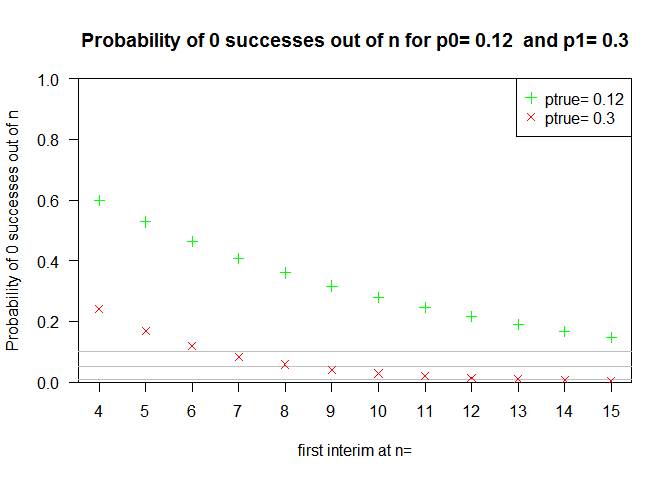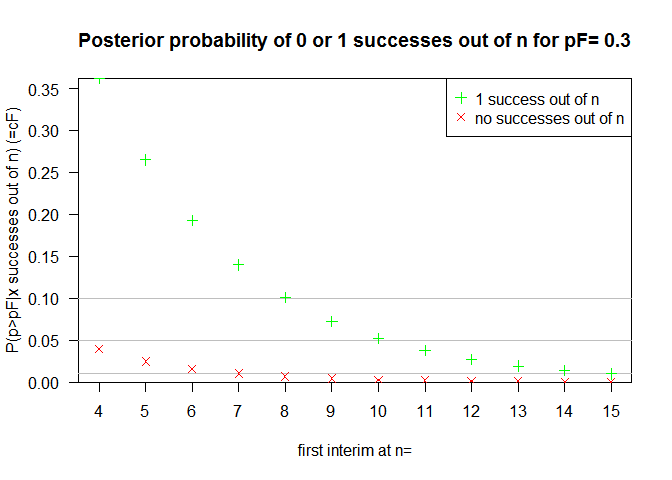# Figure 4

Probability of calling efficacy at final analysis with $$n_\text{final}=20$$ patients as a function of $$c_E$$. Design parameters are $$p_F=0.3, p_E=0.12$$, prior distributions Beta($$p_F,1-p_F$$) and Beta($$p_E,1-p_E$$), $$c_F=0.01$$ and one interim at $$10$$ patients. For $$p_{true}=p_0=0.12$$ this corresponds to type I error, for $$p_{true}=p_1=0.3$$ this corresponds to power.

n=20
interim.at=10
cE=c(7500:9900)/10000

plotBDP2(x = "cE",
y = "PEcall",
n=n, interim.at=interim.at, p0=p0,p1=p1,
pF=pF,cF=cF,pE=pE,cE=cE,
shape1F=shape1F,shape2F=shape2F,shape1E=shape1E,shape2E=shape2E,
col = c("green", "red"))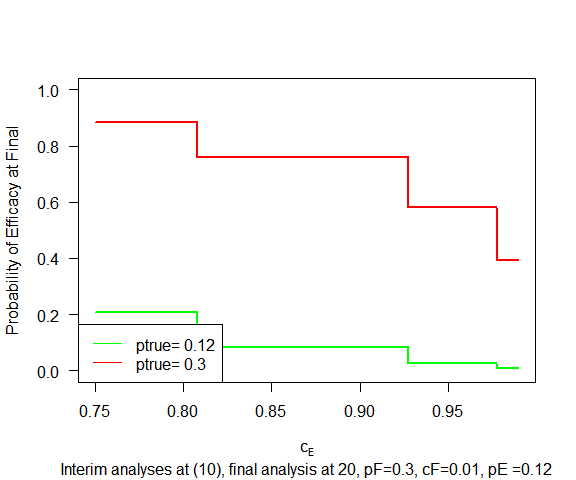# Figure 5

Decision boundaries for futility (in red) and efficacy (in green) for a design with $$c_F = 0.01$$ and $$c_E = 0.9$$. Other design parameters are $$p_F=0.3, p_E=0.12$$, prior distributions Beta($$p_F,1-p_F$$) and Beta($$p_E,1-p_E$$).

n=40
cE=0.9
cF=0.01
plotBDP2(x = "n",
y = "bFbE",
n=n,pF=pF,cF=cF,pE=pE,cE=cE,
shape1F=shape1F,shape2F=shape2F,shape1E=shape1E,shape2E=shape2E,
col=c("red","green"))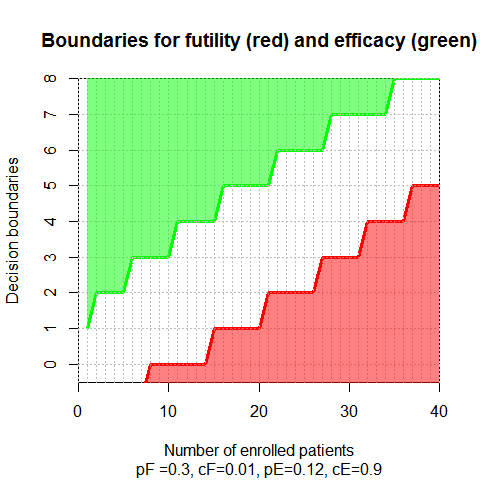# Figure 6

Type I error ($$\text{CumP}_{\text{callE}}$$) and probability of true stopping ($$\text{CumP}_{\text{stopF}}$$) for the uninteresting response rate $$p=p_0=0.12$$ (left panel). Power ($$\text{CumP}_{\text{callE}}$$) and probability of false stopping ($$\text{CumP}_{\text{stopF}}$$) for the target response rate $$p=p_1=0.3$$ (right panel). Other design parameters are $$c_F = 0.01$$ and $$c_E = 0.9$$, prior distributions Beta($$p_F,1-p_F$$) and Beta($$p_E,1-p_E$$), interim analyses every $$10$$ patients.

nvec=c(18:40)
interim.at=c(10,20,30)
ptrue=0.12
plotBDP2(x="n", y="PFstopEcall",
n =nvec, interim.at = interim.at,
pF=pF,cF=cF,pE=pE,cE=cE,ptrue=ptrue,shape1F=shape1F,shape2F=shape2F,shape1E=shape1E,shape2E=shape2E)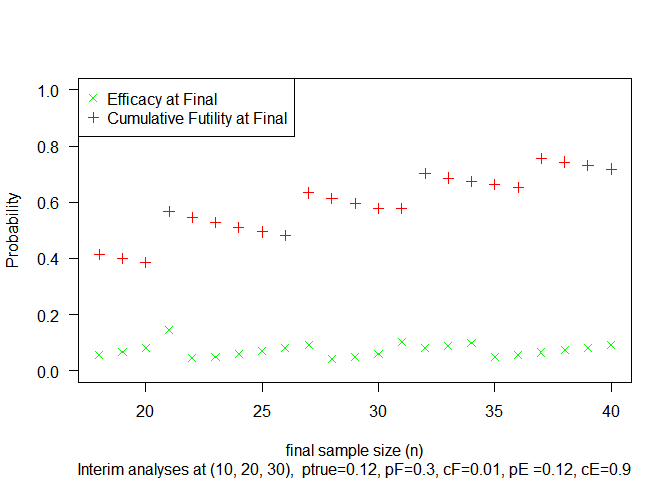ptrue=0.3
plotBDP2(x="n", y="PFstopEcall",
n =nvec, interim.at = interim.at,
pF=pF,cF=cF,pE=pE,cE=cE,ptrue=ptrue,shape1F=shape1F,shape2F=shape2F,shape1E=shape1E,shape2E=shape2E)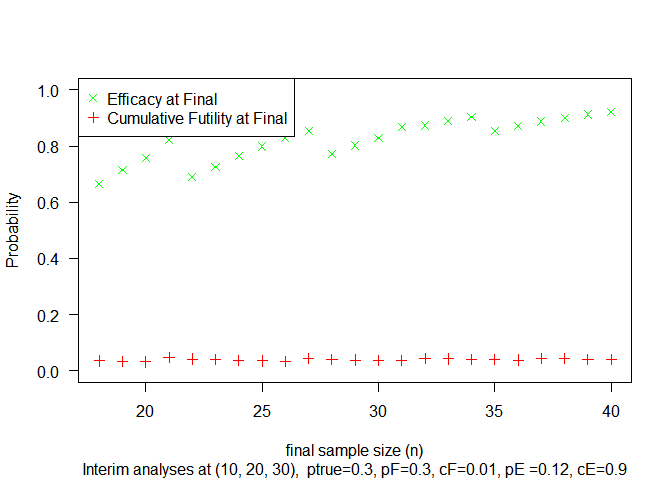# Figure 7

Power function ($$\text{CumP}_{\text{callE}}$$) at $$n_\text{final}= 20$$ (in green) and $$n_\text{final}=30$$ (in red) as a function of $$p$$. Other design parameters are $$p_F=0.3, p_E=0.12$$, prior distributions Beta($$p_F,1-p_F$$) and Beta($$p_E,1-p_E$$), $$c_F=0.01$$ and $$c_E=0.3$$, interim analyses every $$10$$ patients.

n=20
interim.at=10
ptrue=c(0:40)/100
plotBDP2(x = "ptrue", y = "PEcall",
n=n, interim.at=interim.at, ptrue=ptrue,
pF=pF, cF=cF, pE=pE, cE=cE, p0=p0, p1=p1,
shape1F=shape1F, shape2F=shape2F, shape1E=shape1E , shape2E=shape2E ,
col = "green")
n=30
interim.at=c(10,20)
plotBDP2(x = "ptrue", y = "PEcall",
n=n, interim.at=interim.at, ptrue=ptrue,
pF=pF, cF=cF, pE=pE, cE=cE, p0=p0, p1=p1,
shape1F=shape1F, shape2F=shape2F, shape1E=shape1E , shape2E=shape2E ,
col = "red",lty=2,add=TRUE)
abline(v=0.12,col="grey",lty=2)
abline(v=0.3,col="grey",lty=2)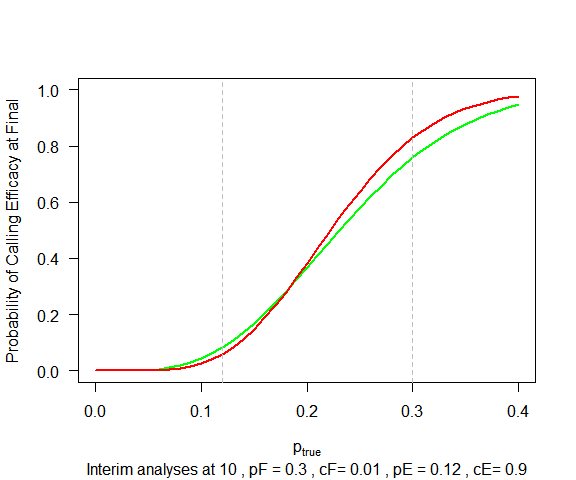# Figure 8

Expected number of patients in the trial for $$n_\text{final}= 20$$ (in green) and $$n_\text{final}=30$$ (in red) as a function of $$p$$. Other design parameters are $$p_F=0.3, p_E=0.12$$, prior distributions Beta($$p_F,1-p_F$$) and Beta($$p_E,1-p_E$$), $$c_F=0.01$$ and $$c_E=0.3$$, interim analyses every $$10$$ patients. Maximal numbers of patients ($$n=20$$ and $$n=30$$, resp.) are shown as dotted lines.

n=30
interim.at=c(10,20)
pvec=c(0:40)/100
interim.at=interim.at[interim.at<n]
plotBDP2(x="ptrue", y="ExpectedNumber",
n=n,interim.at=interim.at,pF=pF,cF=cF,pE=pE,cE=cE,ptrue=pvec,
shape1F=shape1F,shape2F=shape2F,col="red",ylim=c(0,n),cex.lab=1.4)
#> []
#>  10
n=20
interim.at=interim.at[interim.at<n]
plotBDP2(x="ptrue", y="ExpectedNumber",
n=n,interim.at=interim.at,pF=pF,cF=cF,pE=pE,cE=cE,ptrue=pvec,
shape1F=shape1F,shape2F=shape2F,col="green",add=TRUE)
#> []
#>  10

abline(h=20,col="grey",lty=2)
abline(h=30,col="grey",lty=2)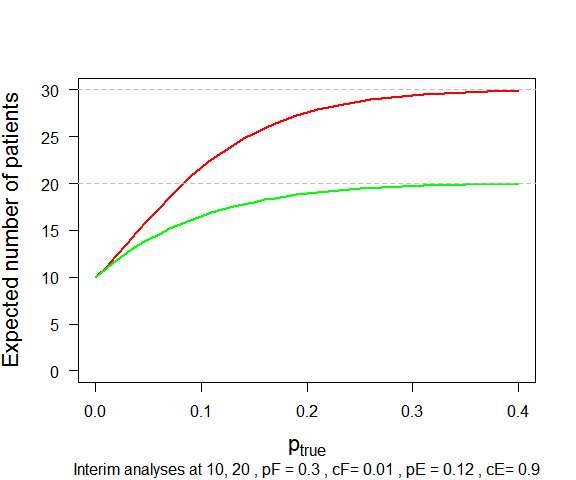# Figure 9

Predictive power (including futility stop) as function of observed responders, evaluated at interim at $$10$$ patients (left panel) or $$20$$ patients (right panel). Final analysis at $$n_\text{final}= 30$$. Other design parameters are $$p_F=0.3, p_E=0.12$$, prior distributions Beta($$p_F,1-p_F$$) and Beta($$p_E,1-p_E$$), $$c_F=0.01$$ and $$c_E=0.3$$

interim.at=c(10,20)
nfinal=30

plotBDP2(x="k",y="PredictivePower",n=nfinal,interim.at = interim.at, cE=cE,cF=cF, pE=pE,pF=pF,
shape1E=shape1E,shape2E=shape2E,shape1F=shape1F,shape2F=shape2F)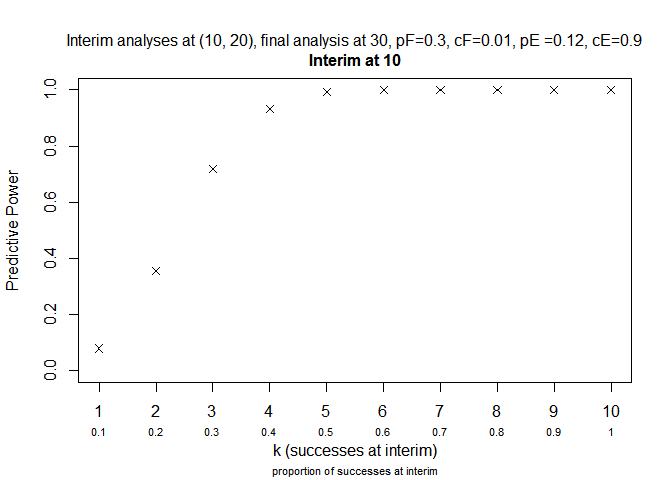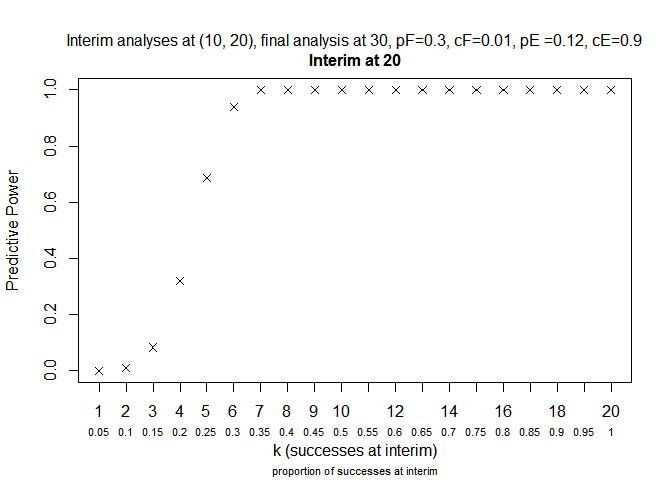# Figure 10

Decision boundaries for futility (in red) and efficacy (in green) for a design with $$c_F = 0.01$$ and $$c_E = 0.9$$ for up to $$100$$ enrolled patients. Other design parameters are $$p_F=0.3, p_E=0.12$$, prior distributions Beta($$p_F,1-p_F$$) and Beta($$p_E,1-p_E$$).

n=100

plotBDP2(x = "n",
y = "bFbE",
n=n,pF=pF,cF=cF,pE=pE,cE=cE,
shape1F=shape1F,shape2F=shape2F,shape1E=shape1E,shape2E=shape2E,
col=c("red","green"))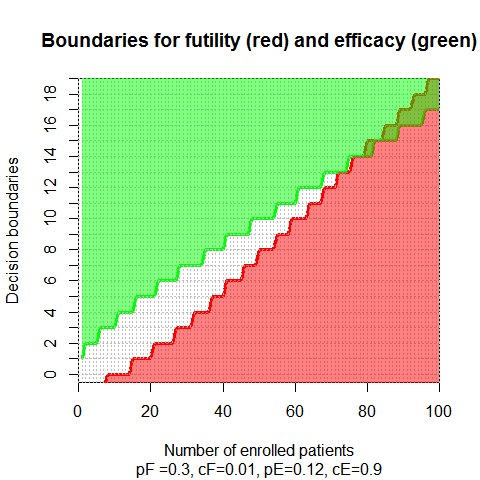## Session info

sessionInfo()
#> R version 3.5.1 (2018-07-02)
#> Platform: x86_64-w64-mingw32/x64 (64-bit)
#> Running under: Windows 7 x64 (build 7601) Service Pack 1
#>
#> Matrix products: default
#>
#> locale:
#>  LC_COLLATE=C                    LC_CTYPE=German_Germany.1252
#>  LC_MONETARY=German_Germany.1252 LC_NUMERIC=C
#>  LC_TIME=German_Germany.1252
#>
#> attached base packages:
#>  stats     graphics  grDevices utils     datasets  methods   base
#>
#> other attached packages:
#>  BDP2_0.1.3    shinyBS_0.61  shiny_1.1.0   rmarkdown_1.9
#>
#> loaded via a namespace (and not attached):
#>   Rcpp_0.12.16    digest_0.6.15   later_0.7.2     rprojroot_1.3-2
#>   mime_0.5        R6_2.2.2        xtable_1.8-2    backports_1.1.2
#>   magrittr_1.5    evaluate_0.10.1 stringi_1.1.7   promises_1.0.1
#>  tools_3.5.1     stringr_1.3.0   httpuv_1.4.3    yaml_2.1.19
#>  compiler_3.5.1  htmltools_0.3.6 knitr_1.20